The definition of rate in a chemical reaction

Rate in chemistry is defined like rate in other scientific fields. Rate is the change in property per unit time. An everyday example is speed which is the change of position divided by travel time.

Likewise, the rate of a chemical reaction is defined as the change in concentration of a substance per unit time.

Considering the reaction,

N2O4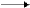2NO2

the rate of decomposition of   N2O4   is given by: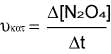the rate of formation of NO2 is given by: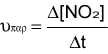If we alter these expressions by replacing the words “decomposition” and “formation” with the word “modification”, the rate of modification of N2O4 would be: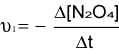and the rate of modification of NO2 would be: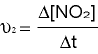The signs - and + in the two last equations imply that  N2O4 is decomposed and  NO2 is produced.
As far as the rate of a reaction is concerned, it should be defined from the modification of one of the components of a substance per unit time.
In the former reaction we observe that for each mol of N
2O4 that is decomposed, 2 moles of NO2 are formed.
In order to equate the rates of formation and decomposition, we have to divide the rate of modification of NO2 by the factor of 2, therefore in the reaction
N
2O42NO   the following definition of the rate results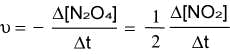If we consider the general type reaction,

aA + bBcC + dD      the rate is given by: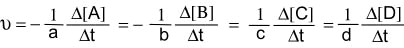under the condition that one of the coefficients a,b,c and d is unit.